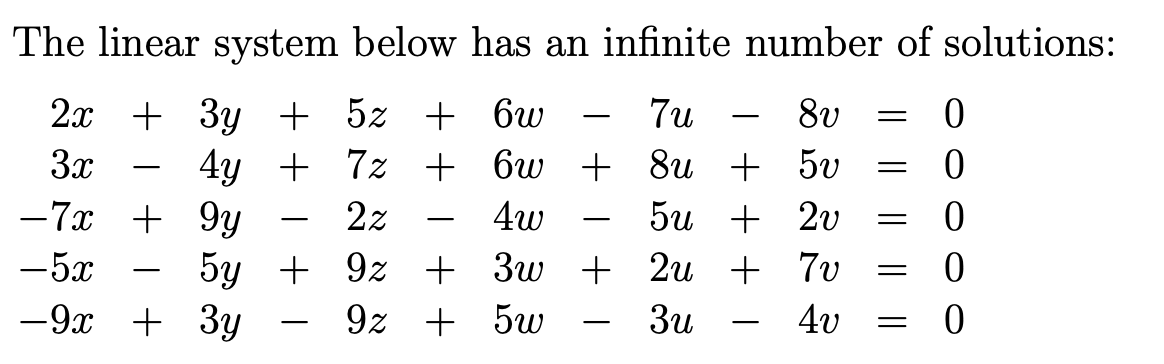# (Solved): True Or False. Justify Your Answer.(Without Reducing To Echelon Form)...

True or False. Justify your answer.(Without reducing to echelon form)The linear system below has an infinite number of solutions: 2x + 3y + 5z + 6 â€“ 7 â€“ 8v = 0 3x â€“ 4y + 7 + 6w + 8 + 50 = 0 -7x + 9y â€“ 2z - 4w - 50 + 20 = 0 -5x - 5y + 92 + 3w + 2 + 70 = 0 -9x + 3y - 92 + 5w - 3u - 40 = 0

We have an Answer from Expert